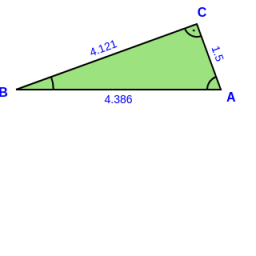# Calculate 60423

In a right triangle RST with a right angle at the vertex T, we know the lengths of two sides: s = 7.8 cm and t = 13 cm; calculate the third party r.

r =  10.4 cm

### Step-by-step explanation:Did you find an error or inaccuracy? Feel free to write us. Thank you!

Tips to related online calculators
Pythagorean theorem is the base for the right triangle calculator.

#### Level of the problem:

We encourage you to watch this tutorial video on this math problem: